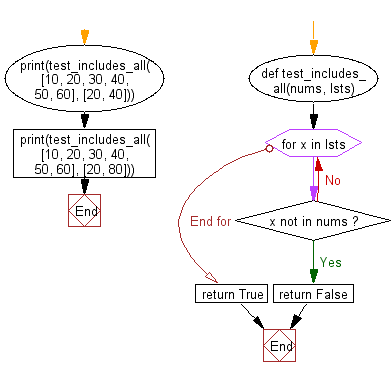﻿ Python: Check if all the elements in values are included in another given list - w3resource# Python: Check if all the elements in values are included in another given list

## Python List: Exercise - 260 with Solution

Write a Python program to check if all the elements of a list are included in another given list.

• Check if every value in lsts is contained in nums using a for loop.
• Return False if any one value is not found, True otherwise.

Sample Solution:

Python Code:

``````def test_includes_all(nums, lsts):
for x in lsts:
if x not in nums:
return False
return True
print(test_includes_all([10, 20, 30, 40, 50, 60], [20, 40]))
print(test_includes_all([10, 20, 30, 40, 50, 60], [20, 80]))
```
```

Sample Output:

```True
False
```

Flowchart:## Visualize Python code execution:

The following tool visualize what the computer is doing step-by-step as it executes the said program:

Python Code Editor:

Have another way to solve this solution? Contribute your code (and comments) through Disqus.

What is the difficulty level of this exercise?

Test your Python skills with w3resource's quiz

﻿

## Python: Tips of the Day

Floor Division:

When we speak of division we normally mean (/) float division operator, this will give a precise result in float format with decimals.

For a rounded integer result there is (//) floor division operator in Python. Floor division will only give integer results that are round numbers.

```print(1000 // 300)
print(1000 / 300)```

Output:

```3
3.3333333333333335```